TDR of connectors

HOME / Applications / TDR of connectors
WEBINAR
How to use HFWorks to design a MIMO antenna system for wireless communication
Thursday, January 20, 2022
Time
SESSION 1
SESSION 2
CET (GMT +1)
03:00 PM
08:00 PM
EST (GMT -4)
09:00 AM
02:00 PM
Used Tools:Description

A coaxial cable with discontinuities (figure 1) is analyzed using the Time-Domain Reflectometry (TDR) solution of the S-parameter module of HFWorks. This is done by sending a pulse signal through the entry port of the cable, and reviewing the reflection produced by the other end of the cable. The shown cable model has three sections separated by discontinuous transitions.

The TDR module can determine the impedance through the path of propagation of the pulse between the tow ports. The designer can then understand where the reflections occur, and determine what may have caused them using some TDR background knowledge.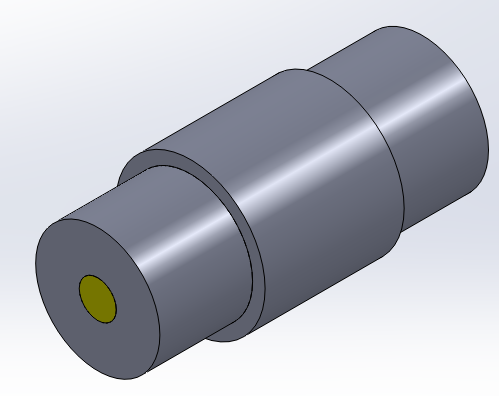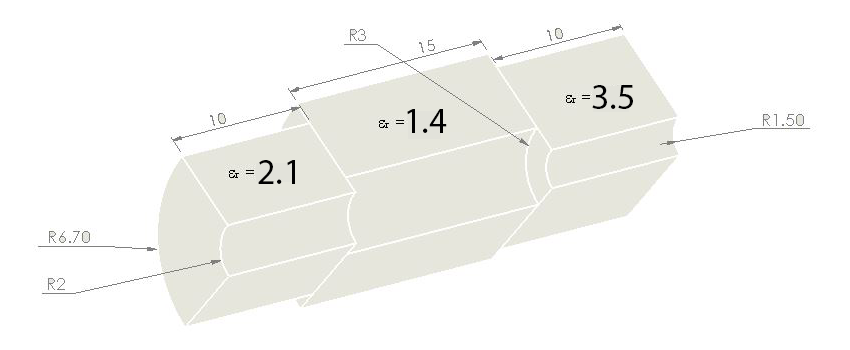Figure 1 - Coaxial cable with discontinuities (Top) with dimensions (Bottom)

Simulation

Simulating this discontinuous coaxial cable portion in an S-parameters-study in HFWorks enables us to investigate its behavior in the frequency domain  (through its Scattering Parameters) as well as its reflectometry properties in time domain (through the TDR simulation found below the Scattering Parameters simulation settings). Switching the signals expressions to time domain from expressions outputted by FEM in the frequency domain requires an IFFT on a number of samples of frequencies (at least two frequencies); Thus, a TDR simulation is not allowed for a single-frequency S-parameters simulation in HFWorks.

As a first step, we perform an S-parameter simulation of the structure, in order to get an idea about the behavior of the structure in the frequency domain. The results of the simulation can be seen in Figure 3. The reflection coefficient S11 reaches more than -25 dB at 5 GHz.. The results of this frequency domain analysis will serve as the input of an IFFT before running the TDR analysis.

Solids and Materials

Figure 1 shows a three parts assembly or structure with similar shapes but slightly different dimensions and relative permittivities. it is from these differences that originate the discontinuities we seek. The predefined material for these blocks or bodies has a relative permittivity of 2.1 for the part in the middle and 3.5 for the lateral ones. When injected into the algorithms calculations, these parameters make the model heterogeneous and thus discontinuities occur in the expressions of the electrical parameters (Voltage, Current, Impedance, Excitation). The plots of these expressions are the key features for the investigation of any transmission model's internal structure.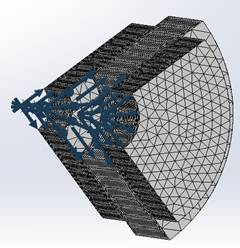The materials have all isotropic permittivity type. Thus, the permittivity parameter is a scalar (For orthotropic material, the permittivity is a 3 by 3 matrix).

In this example, we suppose that we have a Pure TEM propagation; so the check-box "Pure TEM" in the first port is active. Maxwell's equations state that in order to get TEM propagation in  a hallow waveguide (single conductor), we must have a second conductor (signal boundary condition); otherwise, we would have the trivial solution (zero electric field) for Maxwell's equation. Therefore, we assign a signal boundary condition besides the TEM ports. Using boundary conditions along with symmetry (PECS or PMCS) reduces the time of calculations and makes it easier for the simulator to give more realistic results since the contribution of the symmetric portions of the model are automatically taken into account. Considering the electric field direction regarding a face or a plane, we can tell whether we need a magnetic or electric boundary.

Meshing

The mesher of HFWorks calculates the average mesh element size based on the applied mesh controls specified by the user; Then, volume meshing is performed. The mesh elements have smaller size when we approach the surfaces of the mesh control: in our case the inner wire of the coaxial cable. We can also specify the mesh growth rate (set to 1.4 by default).

Results

As mentioned within the beginning of this report, HFWorks uses the FEM solver which runs the calculations of the Maxwell's equation solution in the frequency domain. Thus, to calculate the reflected waves' expressions, an IFFT is applied to the different fields frequency-domain expressions. These expressions will be the means of calculating impedance, voltage and current measured at the considered port. The following figure illustrates the S11 plot in the frequency range 0.625 GHz to 5 GHz. This frequency window should be large enough to compute the IFFT of the different signals.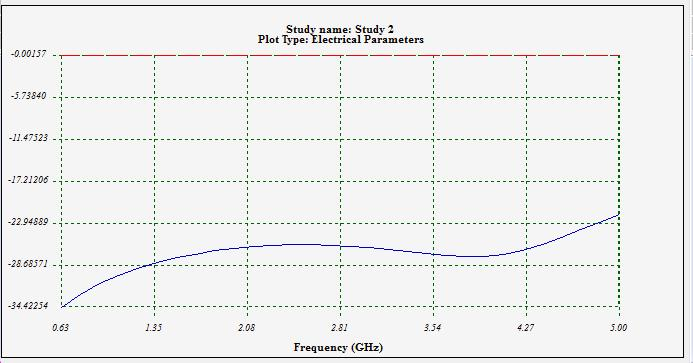Figure 3 - Reflection coefficient and insertion loss

We can view the electric field distribution at different frequencies and animate them by varying the omega-T phase. In this figure, we show two spots of that distribution at different omega-T phases.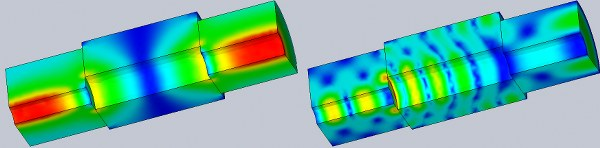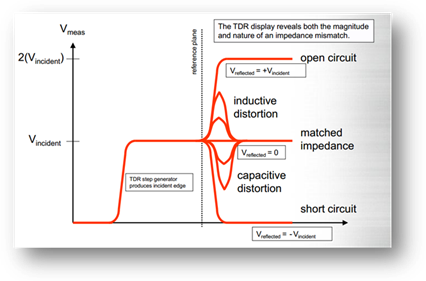Figure 4 - Distance To Fault with impedance information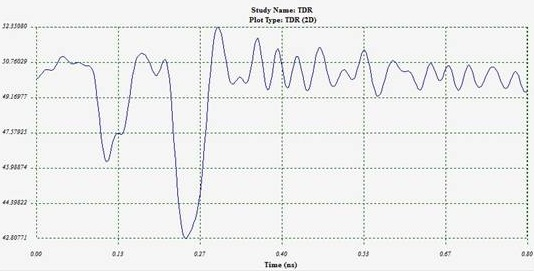Figure 5 - Impedance seen from Port 1

 below imposes a constraint on the system's resolution.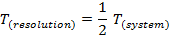Locating Mismatches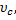For transmission lines with air dielectric. For the general case, where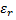is the dielectric constant: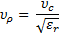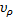= velocity of propagation ; T = transit time from monitoring point to the mismatch and back again.

References

[1 ]  Time Domain Reflectometry (TDR) and S-parameters: Advanced Measurements …not only Signal Integrity - July2009

[2 ]  Tektronix, enabling innovation : TDR Impedance Measurements: Foundation for Signal Integrity

[3 ]  Time Domain Reflectometry Theory Application Note 1304-2- Agilent Technologies